movie

Play recorded movie frames

Description

example

movie(M) plays the movie frames in array M once. To create a movie frame from the current figure or axes, see getframe.

example

movie(M,n) plays the movie n number of times. You can specify n as a numeric array, where the first element of the array specifies the number of times to play the movie, and the remaining elements make up a list of frames to play consecutively in the movie.

example

movie(M,n,fps) specifies the number of frames per second fps at which to play the movie.

example

movie(h,...) plays the movie centered in the figure or axes identified by the figure or axes object h. Specifying the figure or axes enables MATLAB® to fit the movie to the available size.

example

movie(h,M,n,fps,loc) plays the movie at the position specified by a four-element array loc in the current figure.

Examples

collapse all

Use the getframe function in a loop to record frames of the peaks example function, then play the movie frames once.

Create a figure object h. Initialize the surface plot of the peaks function Z. Customize the figure axes.

h = figure;
Z = peaks;
surf(Z)
axis tight manual
ax = gca;
ax.NextPlot = 'replaceChildren';

Preallocate a 40-element array M to store the movie frames.

loops = 40;
M(loops) = struct('cdata',[],'colormap',[]);

For each iteration of j, capture each plot of function X as an individual frame. Store the frame in M.

Set the 'Visible' property of the figure object to 'off' to hide the surface plots while calculating X.

h.Visible = 'off';
for j = 1:loops
X = sin(j*pi/10)*Z;
surf(X,Z)
drawnow
M(j) = getframe;
end

Set the 'Visible' property of the figure to 'on' and play the movie in M once.

h.Visible = 'on';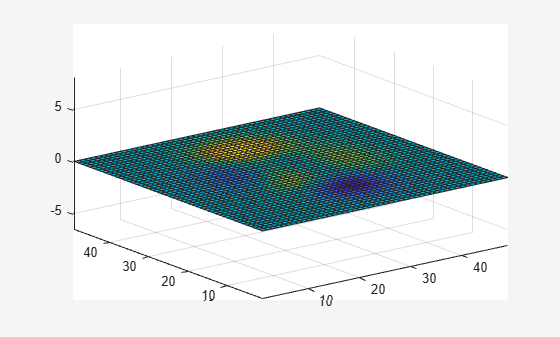movie(M);

View the figure plots while they are calculated. Play the movie array once.

Create a figure object h and initialize the surface plot. Customize the figure axes.

h = figure;
Z = peaks;
surf(Z)
axis tight manual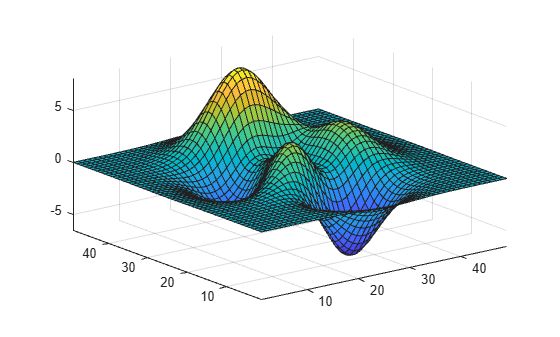ax = gca;
ax.NextPlot = 'replaceChildren';

Preallocate a 40-element array M to store the movie frames.

loops = 40;
M(loops) = struct('cdata',[],'colormap',[]);

Capture each plot of function X as an individual frame and store them in M.

for j = 1:loops
X = sin(j*pi/10)*Z;
surf(X,Z)
drawnow
M(j) = getframe;
end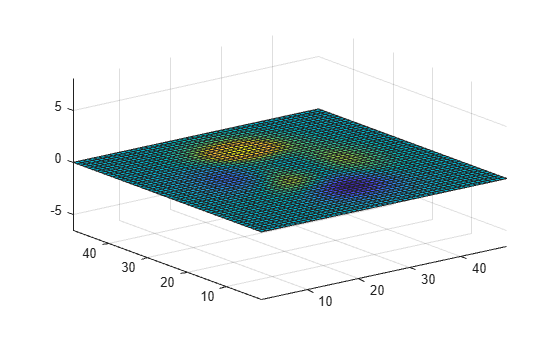Play the movie in M once.

movie(M);

Play four frames of the movie array twice.

Create a figure object h and initialize the surface plot. Customize the figure axes.

h = figure;
Z = peaks;
surf(Z)
axis tight manual
ax = gca;
ax.NextPlot = 'replaceChildren';

Preallocate a 40-element array M to store the movie frames.

loops = 40;
M(loops) = struct('cdata',[],'colormap',[]);

Capture each plot of function X as an individual frame and store them in M.

Set the 'Visible' property of the figure object to 'off'.

h.Visible = 'off';
for j = 1:loops
X = sin(j*pi/10)*Z;
surf(X,Z)
drawnow
M(j) = getframe;
end

Set the 'Visible' property of the figure to 'on'. Play the first, fifteenth, twenty-third, and thirty-sixth frames in the movie in consecutive order twice. Set the movie to play 12 frames per second.

h.Visible = 'on';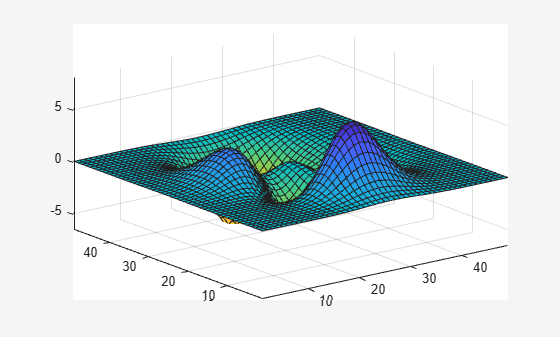movie(M,[2 1 15 23 36],12);

Specify the number of frames per second at which to play the movie.

Create a figure object h and initialize the surface plot. Customize the figure axes.

h = figure;
Z = peaks;
surf(Z)
axis tight manual
ax = gca;
ax.NextPlot = 'replaceChildren';

Preallocate a 40-element array M to store the movie frames.

loops = 40;
M(loops) = struct('cdata',[],'colormap',[]);

Capture each plot of function X as an individual frame and store them in M.

Set the 'Visible' property of the figure object to 'off'.

h.Visible = 'off';
for j = 1:loops
X = sin(j*pi/10)*Z;
surf(X,Z)
drawnow
M(j) = getframe;
end

Set the 'Visible' property of the figure to 'on'. Play the movie once at 6 frames per second.

h.Visible = 'on';movie(M,1,6);

Play the movie frames in the bottom left corner of the figure.

Create a figure object h and initialize the surface plot. Customize the figure axes.

h = figure;
Z = peaks;
surf(Z)
axis tight manual
ax = gca;
ax.NextPlot = 'replaceChildren';

Preallocate a 40-element array M to store the movie frames.

loops = 40;
M(loops) = struct('cdata',[],'colormap',[]);

Set the 'Visible' property of the figure object to 'off'. Capture each figure in handle h as an individual frame and store them in M.

h.Visible = 'off';
for j = 1:loops
X = sin(j*pi/10)*Z;
surf(X,Z)
drawnow
M(j) = getframe(h);
end

Set the 'Visible' property of the figure to 'on'. Play the movie in M once at 12 frames per second and with an offset of 30 pixels in the x and y directions. Specify handle h to play the movie in the current figure.

h.Visible = 'on';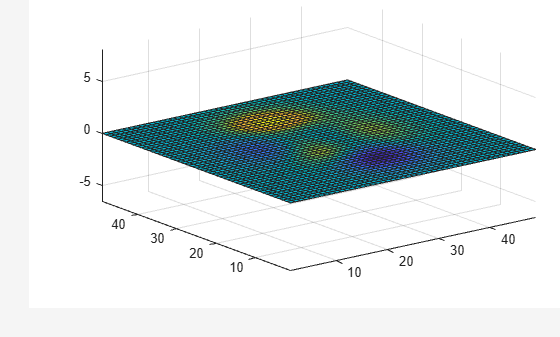movie(h,M,1,12,[30 30 0 0]);

Input Arguments

collapse all

Array of movie frames, specified as an array of structures, where each structure contains the image data captured in the current figure or axes as they appear on the screen. Each row in M corresponds to one movie frame.

You can use the getframe function to capture and store the image data as a structure with the fields cdata and colormap. For more information on the cdata image property and the colormap object, see Image Properties and colormap.

Number of times to play movie in the figure, specified as a numeric array. If you do not specify n, movie will only play the movie once. If n is negative, each cycle is shown forward then backward.

If you specify n as a vector, the first element is the number of times to play the movie, and the remaining elements make up a list of frames to play in the movie.

For example, if M consists of four movie frames and n is an array with elements n = [10 4 4 2 1], then movie plays the movie in M ten times, and the movie consists of the fourth frame, followed by the fourth frame again, followed by the second frame, and finally the first frame.

Frames per second, specified as a numeric scalar. If you do not specify fps, movie will play 12 frames per second. If your machine cannot achieve the speed that you specify as fps, it will play the movie as fast as possible.

Graphics object handle, specified as an axes handle or a figure handle. The default handle of h is gca, which returns the current axes or chart for the current figure. For more information on the gca function, see gca.

If you want to play the movie in the figure instead of the axes, specify the figure handle (or gcf) as the first argument: movie(figure_handle,...).

Location vector, specified as a four-element numeric array, [x y 0 0], where the lower left corner of the movie frame is anchored at the coordinates specified by the first two elements in the array. The coordinates specified in loc are in relation to the lower left corner of the figure or axes specified by handle h, and are in units of pixels. The movie function ignores the last two elements of loc.

Tips

• The movie function uses a default figure size of 560-by-420 and does not resize figures to fit movies with larger or smaller frames. To accommodate other frame sizes, you can resize the figure to fit the movie.

• Buffering the movie places all frames in memory. As a result, on Microsoft® Windows® and perhaps other platforms, a long movie (on the order of several hundred frames) can exhaust memory, depending on system resources. In such cases an error message is issued:

??? Error using ==> movie
Could not create movie frame

You can abort a movie by typing Ctrl-C.